info@biomedres.us   +1 (502) 904-2126   One Westbrook Corporate Center, Suite 300, Westchester, IL 60154, USA   Site Map

Impact Factor : 0.548

# Anything Important That You Should Know About Mixers Volume 7 - Issue 4

• Ericsson Telecom Hungary Ltd., Hungary

Received: July 20, 2018;   Published: August 06, 2018

*Corresponding author: János Ladvánszky, 1117 Budapest, Magyar tudósok körútja 11, Hungary

DOI: 10.26717/BJSTR.2018.07.001537

## Abstract

Our recent investigations on mixers have been overviewed: Comparison, microwave mixer design and adjustment of the conversion loss and the third order intercept point to any prescribed values.

Keywords: Nonlinear Circuits, Mixers, Comparison, Microwave Mixer Design

## Introduction

Goal of this overview paper is to introduce mixer circuits to a broader range of readers. Instead of obtaining a detailed review of literature, we start from definitions and qualitative operation. Then summary of a comparison of six diode and six transistor mixers will follow. The most important Section is about investigation of possible limits of critical large signal properties. A mixer [2-4] is a three-port device. Technical terms like “port” are explained in . A mixer has two input and one output ports. One of them is the RF (radio frequency) input, another one is the LO (local oscillator) input, and another one is the IF (intermediate frequency) output. Function of a mixer in a more complex circuit environment like a radio, is to shift RF input frequency by the frequency of the LO to formulate IF. Down mixing means that the RF frequency is converted down into a much lower IF. This step is necessary because filtering can be more effective at lower frequency. In order to realize this function, the mixer should be nonlinear, time invariant  or linear, time variant . The simplest linear, time variant circuit is a switch. In practice, both can be observed simultaneously. The difference between them is that IF signal of a nonlinear, time invariant circuit consists of infinite number of spectral lines, when the nonlinearity is not polynomial, while that of a linear, time variant circuit contains only finite number of spectral lines.

Intermodulation [2-4] is an unwanted property of nonlinear circuits. That means, if we apply two RF signals, not only the first harmonic of the RF signals, but also higher harmonics are shifted. Expressing this fact by a formula, ωIF=mωRF1+nωRF2−ωLOwhere m,n=±1,±2… Order of intermodulation is |m|+|n|Thus, for example, frequencies of third order intermodulation are 2ωRF1RF2−ωLO, 2ωRF1RF2−ωLO, -2ωRF1RF2−ωLO, ωRF1RF2−ωLO, ωRF1-2ωRF2−ωLO,-ωRF2+2ωRF2−ωLO. If the frequency difference between the two RF inputs is low, then the useful signal 1RFLOωω−is near to the intermodulation at 122RFRFLOωωω−−. That means the intermodulation disturbs the useful signal and therefore it should be kept at as low amplitude as possible. Intermodulation is characterized by the intercept point IPn where n is the order of intermodulation. IIPn and OIPn are the input and output intercept points. The definition of the output intercept point is the output power level where the straight lines fit to the RF power dependence of the fundamental and the intermodulation IF powers, intersect each other. Another important mixer characteristic is the conversion loss. That is the difference in dB between the IF output and the corresponding RF input power. Some more mixer characteristics are isolations between ports. These are differences in dB between components of the the same frequency at different ports.

Note: *None of them needs DC supply except the four-quadrant multiplier, ±12 V 30 mA.

Table 2: Comparison of Analysis and Measurement.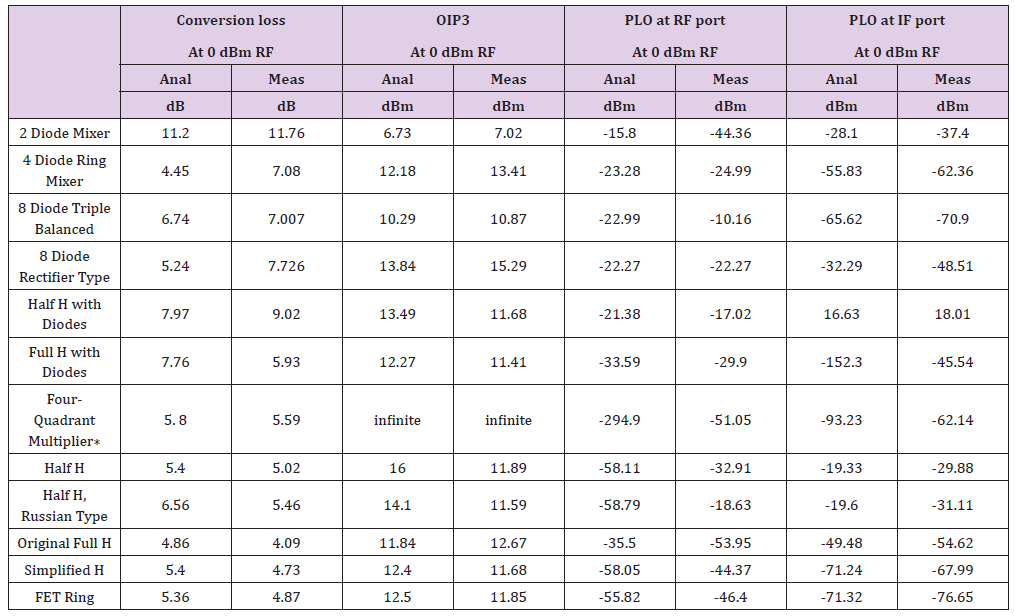Figure 1:A: Six diode mixers and

B: Six transistor mixers.In Figure 1, six diode and six transistor mixer realizations are shown . Based on (Tables 1 & 2), our modification of the fourquadrant multiplier is the best one. Schematic diagrams are found in .

### Microwave Mixer Design 

These investigations start with the discovery of a new mixer topology that we call as half H mixer. After transformation of the schematic diagram, we reach a MHz range mixer topology that is suitable for microwave realization. Details of the microwave realization have also been given. Let us start with the full H diode mixer . The skeleton of the mixer circuit diagram is an uppercase H. Diodes are found in the vertical branches. Cut the diagram along the horizontal symmetry axis and leave the circuit components below the axis. The remaining part is the half H mixer. As full H comprises four diodes, only two of them are necessary to build a half H (Figure 2). Then the circuit undergoes a series of transformations . A Capacitive LO feed replaces transformers S3 and S4. A series resonator is inserted between the IF port and the transformer S5. The LO port is directly connected to the transformer S5. The structure is realized at microwaves (Figure 3). For convenience, the rat-race hybrid is realized in oval form (Figure 4). The picture and the characteristics are shown in Figures 5 & 6. Critical part of the design procedure was modelling of the microwave diodes. A novel diode model has been provided .

Figure 6:Microwave characteristics. Component powers at the IF port. Blue triangles: fundamental, violet squares: third order component, brown diamonds: LO power at RF port, red crosses: LO power at IF port. Filename: DhalfH3charm9.emp. Measured conversion loss at 0 dBm is 14.13 dB.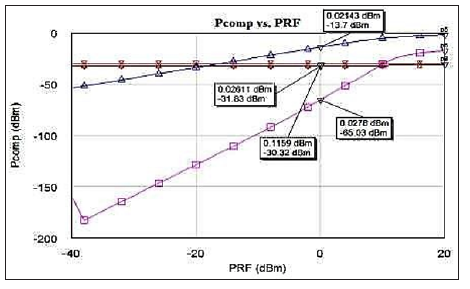### Is There an Upper Limit of the IP3? 

Let us consider the following Figures 7 & 8 about intermodulation products of an unbalanced two-diode mixer .

Figure 7:OIP3 at the IF port, blue triangles. Filename: DhalfH3eharm9.emp. Measured OIP3 at 0 dBm is 8.21 dBm.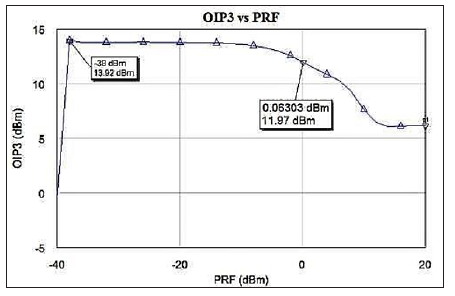Figure 8:Intermodulation vs. input power for the diode half H mixer. Infinite IP3 phenomenon is observed here as well. Notations: Triangles – first order, squares – third order intermodulation products (AWR analysis), circles – measurements.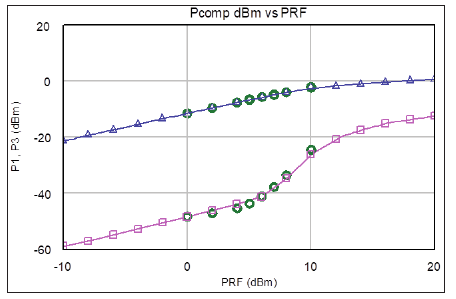Figure 9:Power of the first and the third order intermodulation of a modified Gilbert mixer vs. input power. The two curves can be approximated well by two parallel lines. Parallel means that the point of intersection is at the infinity. Thus, this is called as infinite IP3 phenomenon. Notations: Triangles – first order, squares – third order intermodulation products (AWR analysis), circles – measurements.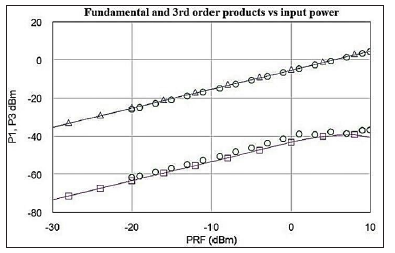In a region of the RF input power, first and third order intermodulation curves are approximately parallel. That means a very large (infinite) OIP3 because lines fit to these parts of the two curves do not intersect with each other. The same phenomenon is shown in other mixers as well, e.g. in a Gilbert mixer (Figure 9). We pointed out that a possible reason of this phenomenon is the inductance in series with the diodes.

We also pointed out that gain factors in a mixer system (Figure 10) can be adjusted to achieve an arbitrary IL and OIP3.

where G and G2 are the voltage gain factors of the pre- and the post-amplifier, respectively. Prime denotes the mixer system, lack of prime denotes the mixer block in the middle. This result proves that there is no theoretical upper bound of IP3, only practical. Practical upper bound is the consequence of the finite IP3 of the pre- and post-amplifiers that we assumed here linear.

## Summary

After introducing the necessary concepts, we presented a comparison between six diode and six transistor mixers. Following this, a design procedure for a 10 GHz mixer has been shown. Finally, we obtained a simple circuit capable of adjusting the conversion loss and the third order intercept point of a mixer to any prescribed values.

## Acknowledgment

Prof. Dr. T. Berceli at the Budapest University of Technology and Economics supported me for several years. From the Optical and Microwave Laboratory he sent to me several talented students for diploma work. One of them was Kristóf Máté Osbáth, who made several mixer measurements for me.